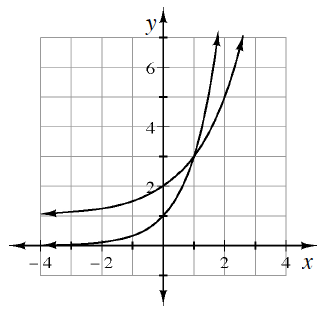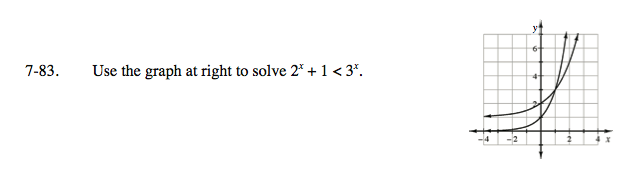Home > INT3 > Chapter 7 > Lesson 7.2.3 > Problem7-83

7-83.
1. Use the graph below to solve 2x + 1 < 3x. Homework Help ✎

2.First decide which curve represents y = 3x. Highlight this curve. For what values of x is your highlighted curve above the other curve?

$x>1$